# MultiTable

## MultiTable(i1,i2,...)(src1, src2, ...)

A MultiTable lets you mix editable and computed values in a single table. Editable cells are based on other variables, each defined as a Table or SubTable. Computed values are based on calculated variables or expressions. When you change an editable cell, you are actually changing the original cell in the source table, not a copy.

It is best practice in Analytica to keep different editable user inputs and computed results in different variables. But, it is often convenient to define part of a user interface that combines editable and computed values, which the MultiTable lets you do.

For example, suppose Startup_cost and Prob_success are both edit tables, indexed by Project. Then the a variable defined as:

Variable Project_inputs := MultiTable(Item)(Startup_cost, Prob_success)

lets you view and edit both Startup_cost and Prob_Success in the same edit table. The MultiTable is indexed by Project, the index common to both Startup_cost and Prob_success, and by Item, which is an additional index that needs to be created.

MultiTable is the inverse of SubTable in that it combines multiple sources into one view, where SubTable selects a particular slice or subset of data to edit.

## Creating a MultiTable

Before you create a MultiTable, you need to create or identify one or more indexes that your data sources will vary over. For example, if you want a different data source to appear in each column of your table, then you'll need a column index, and you'll need to fill in the labels for each column as you define the index.

Next, create a variable to hold your MultiTable and press Ctrl+E to place your cursor in the definition field.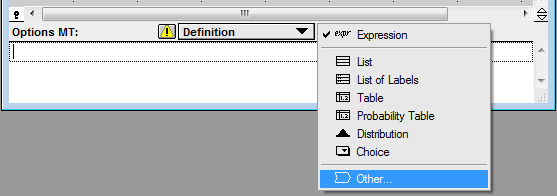In the Object Finder, select the Array library and scroll down to find and select MultiTable. Then, press the Indexes button.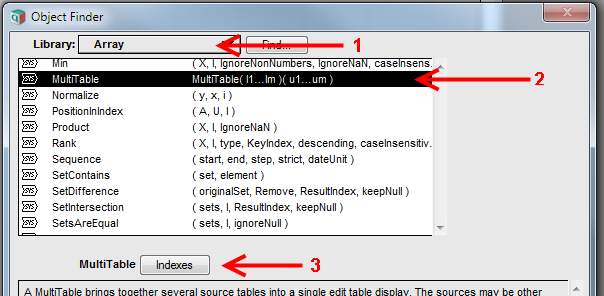Select the indexes that your MultiTable data sources will vary along. These are not the indexes of your data sources themselves.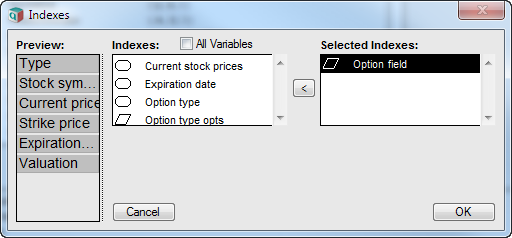The table initially displays the "Meta expressions" view so that you can specify the data sources for the table. The meta-expression selector controls which view you are in.

## Specifying Data Sources

Whenever you need to specify or change which data source should appear in a MultiTable, use the meta-expressions selector to change to meta-expressions view.

Here is an example of meta-expressions.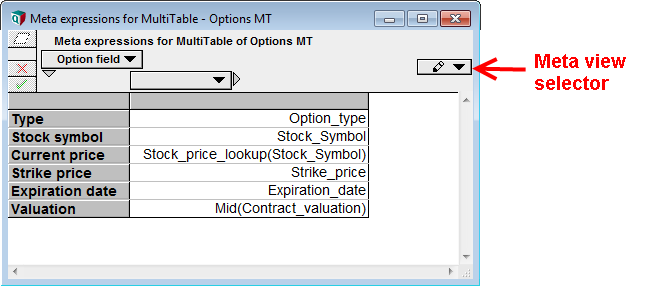In the above meta-expressions view, Option_type, Stock_symbol, Strike_price and Expiration_date are identifiers of other edit tables. These will be editable columns. Stock_price_lookup(Stock_Symbol) is a call to a User-Defined Function, and its computed value will appear in this column. The last cell contains Mid(Contract_valuation), so that the computed mid-value of Contract_valuation will be displayed. When you want the computed value of a variable, you must surround its identifier with Mid in this fashion, otherwise its definition will appear in the cell and will be editable.

### Editable sources

An editable source reflects the contents of another edit table in your model, a slice of an edit table, or the definition of another variable. When a user edits these cells in the multitable, they are actually changing the data in the specified source definition or table. In meta-expression view, an editable source is specified in one of the following ways.

• X : An identifier. The definition of X, or the edit table (or SubTable or MultiTable of X) is depicted.
• X[I=v] : A Slice or Subscript of another table. That slice is depicted in an editable form.

The source can be a scalar variable, an edit table, a SubTable or another MultiTable. Rather arbitrary transformations that be accomplished by nesting SubTables and MultiTables.

### Computed sources

The following are computed expressions, which are evaluated and the resulting value displayed in a non-editable form with a gray background.

• 31.4, "Text": Literal values (numbers, quoted text)
• Mid(X): Displays the computed mid-value of the variable X
• F(...): Any function call other than Slice or Subscript, including calls to User-Defined Functions, are evaluated.
• x + y : An expression, such as one involving arithmetic operations, is evaluated.

Here are a few additional examples:

• Mid(X) : computes and displays the mid-value of X
• Abs(X) : displays the absolute value of the (mid-value) of X
• Mean(X) : computes and displays the mean of X
• 1.2 : displays the number, 1.2, in a non-editable cell
• "Some text" : displays the text, without quotes, in a non-editable cell

A computed expression or computed variable CAN depend on the values of other data sources that appear in the same MultiTable. When you do this, the computed value will change when the user changes the input cells and presses the green check button.

## Viewing the Data

After you have specified the data sources in meta-expression view, change the meta-expression selector to Show Cells to see the actual data.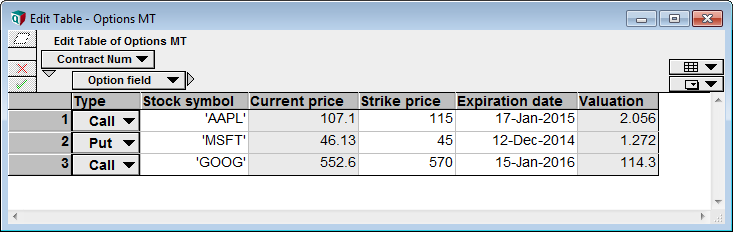### When cells can be edited

You can never edit computed values in a MultiTable

In Browse mode, you can edit data in a variable only if the variable has a user input node. This means that if your MultiTable variable has a user input node, users in Browse mode (including Analytica Cloud Player or Free Edition) can edit all table sources. Otherwise, if one source has a user input node and another does not, users in Browse mode can edit only those cells from the source(s) with the user in.

## Examples

Here is an example of an editable value and computed value in the same table:

 Index Base := ["Decimal", "Hex"]
Variable x := 123
Variable HexConverter := MultiTable(Base)(x, NumberToText(x, "Hexadecimal"))


The edit table displays like this: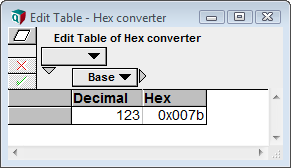The first cell can be edited, and when you change it and press the green check, the hexadecimal value is computed and displayed.

## Dimensionality

The dimensions of the MultiTable content are the union of the indexes from all sources and of the MultiTable index(es).

A MultiTable works best when your sources all have the same dimensionality. When one source does not have an index present in another source, a single cell in the source will map to multiple cells in the MultiTable; hence, you'll see the same value multiple places, and when you change it, multiple cells will change. The source retains its original dimensionality, so if the value doesn't vary along an index in the source, it won't vary along that index is the MultiTable. You may temporarily see a difference in these cells after you first enter a value, but they will become consistent as soon as you press the Green check.

## Number Formats

When number formats are set for the source tables, those formats are used in the corresponding column of the MultiTable.

When a meta-expression is of the form Mid(X), then X's number format is used. For arbitrary expressions, the number format of the MultiTable's own variable is used.

## MultiTable vs. SubTable

A SubTable and MultiTable are very closely related. Each presents a table view of data that is located in one or more different sources. In each case, the table view can contain a mixture of editable and computed cells. They can even be nested in any combination -- i.e., each source a MultiTable can be another MultiTable or another SubTable (in which cases, the real location of the data is two (or more) steps away). There are cases where you can obtain the same table view using either a SubTable or a MultiTable. There are also cases where only one of the two will get you to your desired view (in these cases, it is usually theoretically possible to do it with either, but the wrong choice is excessively complex and inflexible). This brings up the question -- how do you figure out which one you need to use?

SubTable( expr ) accepts a single expression. The expr has precisely the same form as one cell expression in a MultiTable. Thus, one question to ask is: Are you adding a new index (or indexes) to your view that will index the sources? If yes, then you probably want a MultiTable. If no, then you probably want a SubTable.

The following example illustrates this idea (download this example model). You have two source tables, each indexed by Move_num:

Index Move_num := [1, 2, 3, 4, 5]
Variable Whites_move := Table(Move_num)
Variable Blacks_move := Table(Move_num)

The source edit tables contain initially: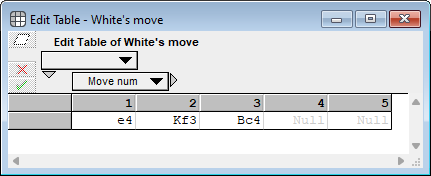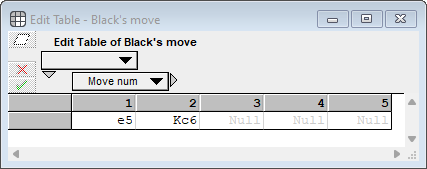In the first variation, you want to combine these into an editable move table as follows: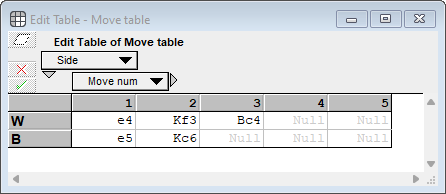In this variation, the index Side indexes the source tables, so therefore, MultiTable is the correct option.

In the second variation, you want to combine these into a linear move sequence as follows: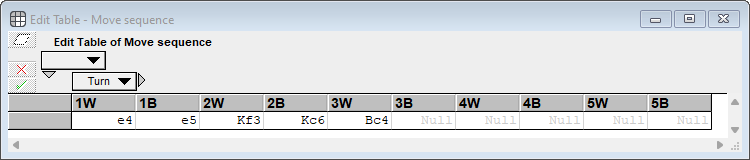This maps the sources to a new index

Index Turn := ['1W', '1B', '2W', '2B', '3W', '3B', '4W', '4B', '5W', '5B' ]

In this case, the index, Turn indirectly indexes the source, but it doesn't directly index the sources. While it is possible to use a MultiTable with the index Turn, you would need to insert a separate expression in all 10 cells. If, in the future, you change the maximum number of moves, if take some work to get the new cells to automatically start with the correct cell default. So for this variation, a SubTable is a much better option than a MultiTable. One way to define the SubTable is as

SubTable( If Mod(@Turn,2)=1 Then Whites_move[Move_num=Move_num_of_turn] Else Blacks_move[Move_num=Move_num_of_turn] )

## History

Introduced in Analytica 4.6. Present in experimental form in Analytica 4.5.